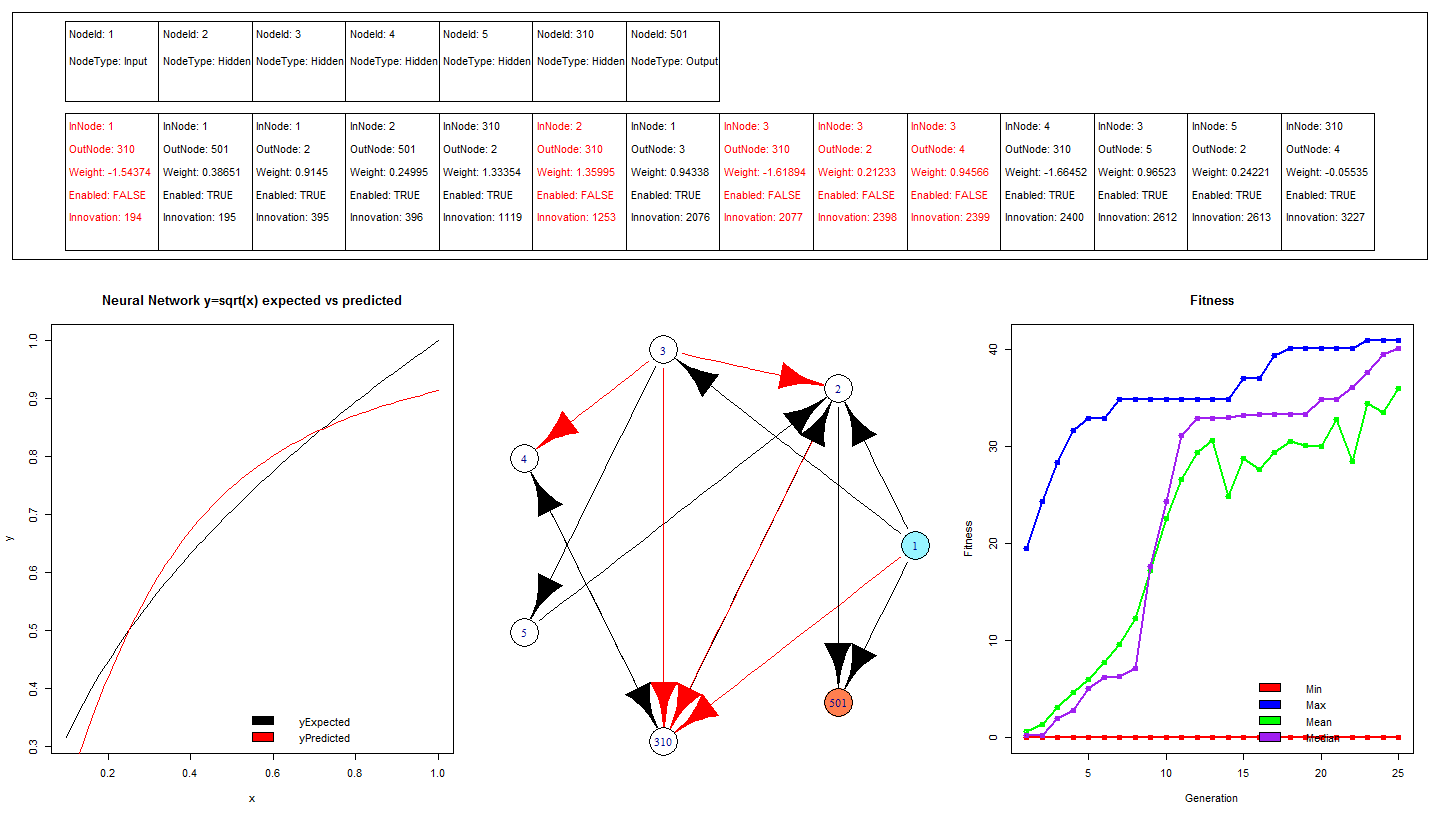RNeat – Square Root Neural Net trained using Augmenting Topologies – Simple Example

A simple tutorial demonstrating how to train a neural network to square root numbers using a genetic algorithm that searches through the topological structure space. The algorithm is called NEAT (Neuro Evolution of Augmenting Topologies) available in the RNeat package (not yet on CRAN).

The training is very similar to other machine learning / regression packages in R. The training function takes a data frame and a formula. The formula is used to specify what columns in the data frame are the dependent variables and which are the explanatory variable. The code is commented and should be simple enough for new R users.The performance of the network can be seen in the bottom left chart of the image above, there is considerable differences between the expected output and the actual output. It is likely that with more training the magnitude of these errors will reduce, it can be seen in the bottom right chart that the maximum, mean and median fitness are generally increasing with each generation.

?View Code RSPLUS
 install.packages("devtools") library("devtools") install_github("RNeat","ahunteruk") #Install from github as not yet on CRAN library("RNeat")   #Generate traing data y = sqrt(x) trainingData <- as.data.frame(cbind(sqrt(seq(0.1,1,0.1)),seq(0.1,1,0.1))) colnames(trainingData) <- c("y","x")   #Train the neural network for 5 generations, and plot the fitness rneatsim <- rneatneuralnet(y~x,trainingData,5) plot(rneatsim)   #Continue training the network for another 5 generations rneatsim <- rneatneuralnetcontinuetraining(rneatsim,20) plot(rneatsim)   #Construct some fresh data to stick through the neural network and hopefully get square rooted liveData <- as.data.frame(seq(0.1,1,0.01)) colnames(liveData) <- c("x")   liveDataExpectedOutput <- sqrt(liveData) colnames(liveDataExpectedOutput) <- "yExpected"   #Pass the data through the network results <- compute(rneatsim,liveData)   #Calculate the difference between yPred the neural network output, and yExpected the actual square root of the input error <- liveDataExpectedOutput[,"yExpected"] - results[,"yPred"] results <- cbind(results,liveDataExpectedOutput,error)   dev.new() layout(matrix(c(3,3,3,1,4,2), 2, 3, byrow = TRUE),heights=c(1,2)) plot(x=results[,"x"],y=results[,"yExpected"],type="l", main="Neural Network y=sqrt(x) expected vs predicted",xlab="x",ylab="y") lines(x=results[,"x"],y=results[,"yPred"],col="red",type="l") legend(x='bottomright', c('yExpected','yPredicted'), col=c("black","red"), fill=1:2, bty='n') plot(rneatsim) plot(rneatsim\$simulation)